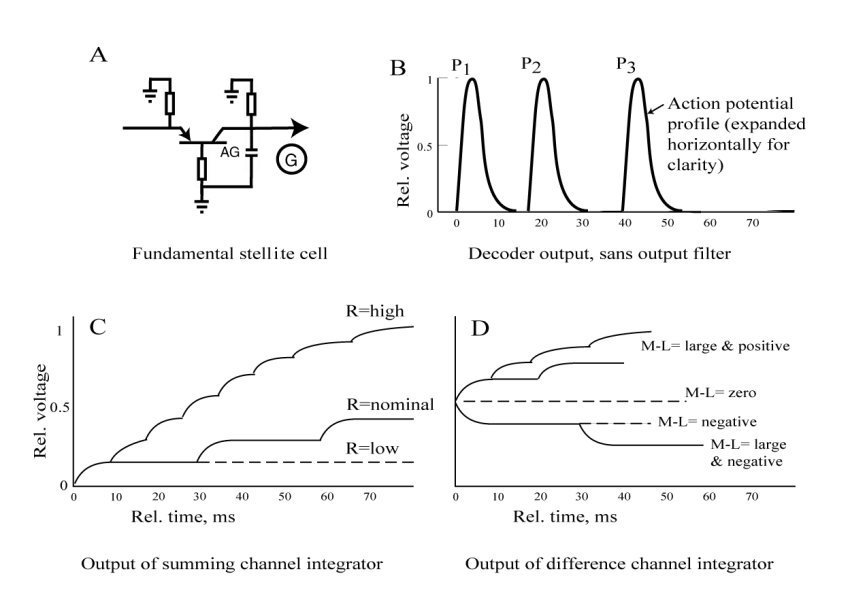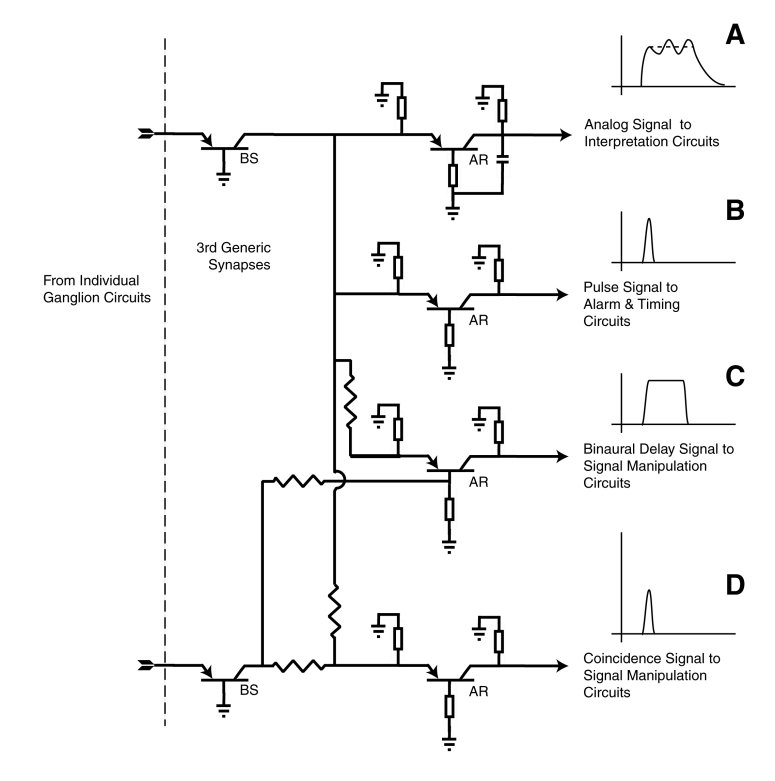...

...

The Neural Code Recovery Circuits

This is the third of three closely associated pages discussing 1) the overall phasic stage (stage 3) of neural signaling , 2) the encoding of analog neural signals as action potentials and the resultant neural code, and 3) the recovery of the original analog signal from the encoded pulse stream (action potential stream).

Last Update:

The term stellite is used as the functional description of the decoding neurons of stage 3. It describes a large number of the morphologically defined stellate neurons and includes a majority of the large neurons of layer IV of the cerebral tissue.

Function is used here in the context of the underlying process of a given neural structure, and not as frequently found in neuroscience texts to describe the purpose of the structure.

The topology of the fundamental neural code recovery circuit

The following figure shows the fundamental neural configuration found in all stellite (signal recovery) neurons in Frame A. This configuration is the same as that used in virtually all neural circuits. However, the values of the parameters are different.

• The impedance in the base (poditic) circuit is so low, the internal feedback due to that impedance is not sufficient to support overall circuit oscillation.
• The capacitor shown at the lower right is so large relative to the axon impedance shown at upper right that the output circuit acts as a low pass filter. As such, it integrates the currents generated by the applied action potentials.
• The applied signal is a phasic signal but the output at G is its time integral, an analog signal.

The recovery of an electrolytically monopolar pulse train

Frame B shows the signals at the axon of the stellite neuron in the absence of the large capacitor. The waveforms are duplicates of the input action potentials. The 1-2 ms wide waveforms are shown widened for pedagogical purposes.

Frame C shows the signals recovered at G for the condition where the input action potential stream is discontinuous. It consists of one or more individual monopulses from an electrolytically monopolar signal channel. The shape of the recovered signal depends on the time-delay between the pulses. At low time-delay, high pulse rates, the signal rapidly reproduces the nominal amplitude of the analog stimulus applied to the ganglion neuron generating the pulses.

The last statement is consistent with the proposal that no computation occurs within the fundamental circuits of stage 3. In the fundamental stellite circuit, the recovered analog signal is a copy of the analog signal applied to a single ganglion neuron.Frame D shows the signals recovered at G for the condition where the input action potential stream is continuous. The output at G achieves an average potential (shown here as 0.5) in the absence of any stimulus to the associated ganglion neuron, which operates in a free-running pulse mode. For a bipolar analog signal causing a reduction in the time delay between pulses, the output rises (with a temporal profile that reflects the time delay interval between the pulses). The ultimate height reflects the amplitude of the original analog signal.

Note the long time required to recover the amplitude of a highly negative modulation of the ganglion neuron (resulting in long time intervals between the action potentials. Meaningful output is delayed considerably. This condition is commonly recognized in the visual system where the difference between the green and red signaling channels (commonly described as the Mid-wavelength minus the Long-wavelength signal. The condition is memorialized in the "mixed highs" form of color television transmission.

The recovery of additonal information from more complex neural code

The previous discussion related to the fundamental stellite decoding circuit. By changing the impedance values of the fundamental circuit only slightly, additional information can be extracted from the ganglion neuron signal stream. The following figure shows four of these modified circuits and their output.

• Frame A shows the fundamental circuit regenerating the analog signal applied to the ganglion neuron.
• Frame B shows the circuit outputing only the initial pulse for use in alarm and timing circuits
• Frame C shows the circuit modified for two inputs. The resulting output is a pulse width proportional to the difference in timing between the two inputs, particularly useful in auditory source location.
• Frame D shows the circuit used as a coincidence circuit comparing two inputs.

All of these output signals are obtained using the same basic stellite circuit with simple impedance value and bias changes.Go to the first page of this subject set, the neural signal projection mechanism.
Go to the second (preceding)page of this subject set, the decoding mechanism for the neural code.